# Ex.12.1 Q2 Algebraic Expressions Solution - NCERT Maths Class 7

## Question

(i) Identify the terms and their factors in the following expressions. Show the terms and factors by tree diagrams.

(a) $$x – 3$$

(b) $$1 + x + x^2$$

(c) $$y – y^3$$

(d) $$5xy^2 + 7x^2y$$

(e) $$– ab + 2b^2 – 3a^2$$

(ii) Identify terms and factors in the expressions given below:

(f) $$–4x + 5$$ (b) $$–4x + 5y$$ (c) $$5y + 3y^2$$

(g) $$xy + 2x^2y^2$$ (e) $$pq + q$$ (f) $$1.2 ab – 2.4 b + 3.6 a$$

(h) $$\frac{3}{4}x + \frac{1}{4}$$ (h) $$0.1 p^2 + 0.2 q^2$$

Video Solution
Algebraic Expressions
Ex 12.1 | Question 2

## Text Solution

(i) Identify the terms and their factors in the following expressions. Show the terms and factors by tree diagrams.

(a) $$x – 3$$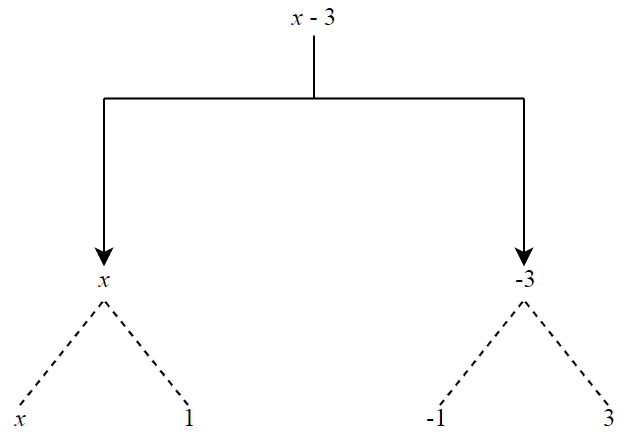Steps:

Term $$= x$$ and Factor $$= 1$$

(b) $$1 + x + x^2$$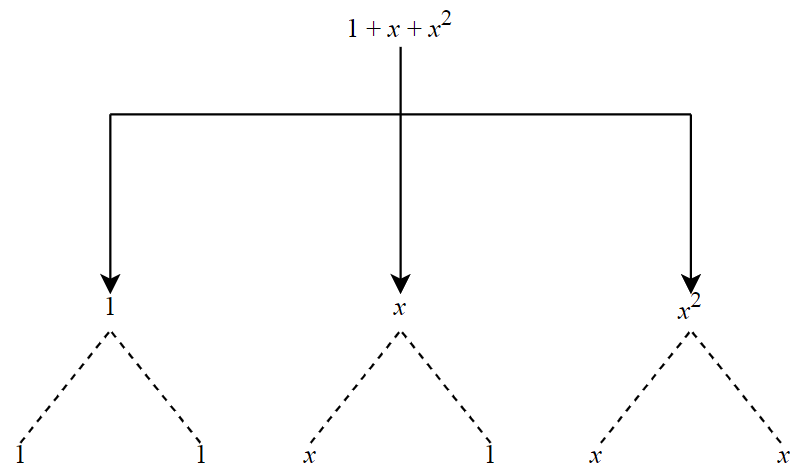Steps:

Term $$= x$$ and Factor $$= 1;$$ Term $$= x^2$$ and Factor $$= 1$$

(c) $$y – y^3$$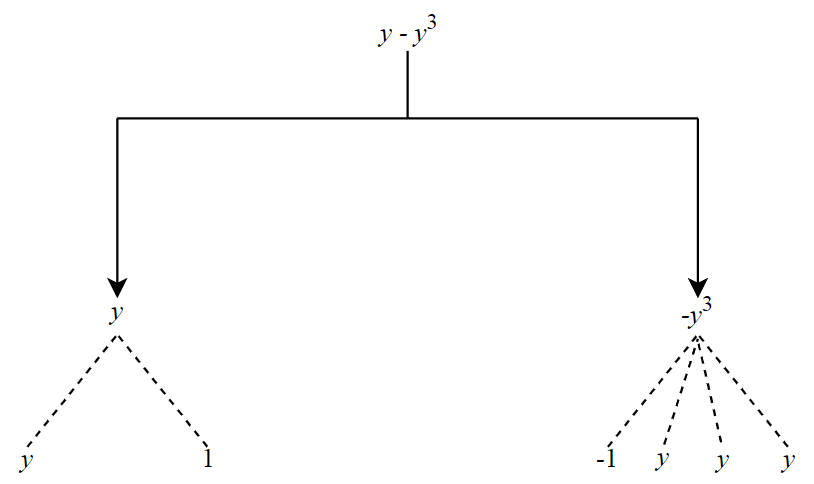Steps:

Term $$= y$$ and Factor $$= 1;$$ Term $$= y^3$$ and Factor $$= –1$$

(d) $$5xy^2 + 7x^2y$$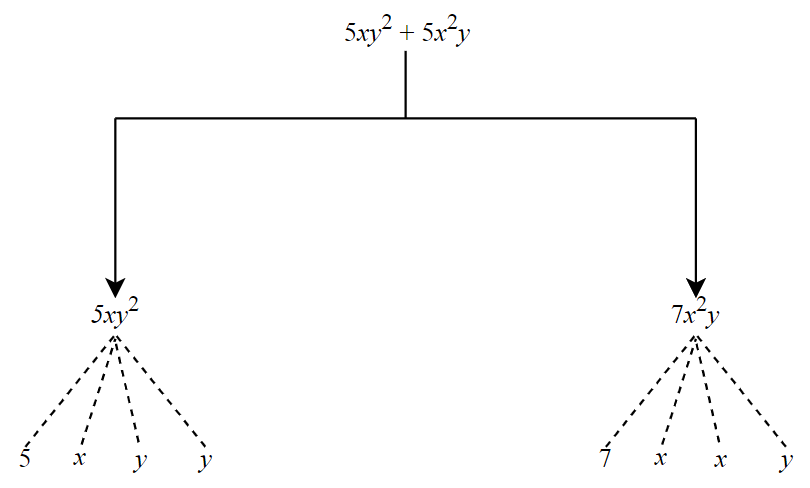Steps:

Term $$= xy^2$$ and Factor $$= 5;$$ Term $$= x^2y$$ and Factor $$= 7$$

(e) $$– ab + 2b^2 – 3a^2$$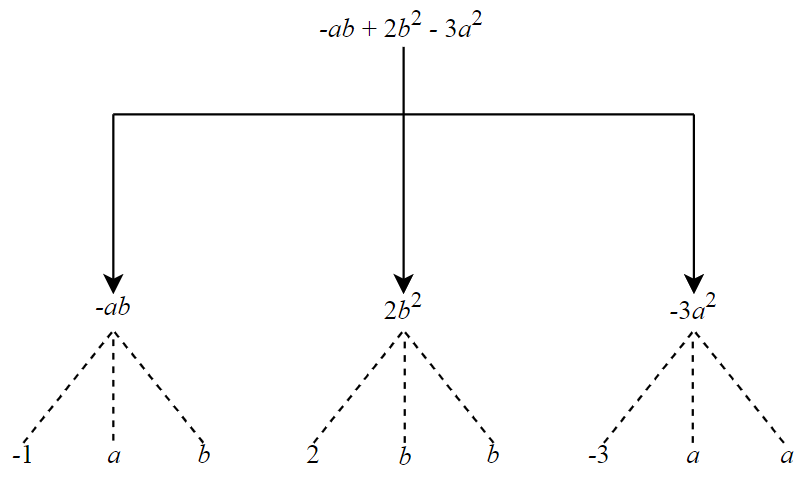Steps:

Term $$= ab$$ and Factor $$= –1;$$Term $$= b^2$$ and Factor $$= 2;$$ Term $$a^2$$ and Factor $$= –3$$

(ii) Identify terms and factors in the expressions given below:

(f) $$–4x + 5$$ (b) $$–4x + 5y$$ (c) $$5y + 3y^2$$

(g) $$xy + 2x^2y^2$$ (e) $$pq + q$$ (f) $$1.2 ab – 2.4 b + 3.6 a$$

(h) $$\frac{3}{4}x + \frac{1}{4}$$ (h) $$0.1 p^2 + 0.2 q^2$$

 S.No. Expression Term Factors a) $$–4x + 5$$ $$-4x$$ and $$5$$ $$-4, x$$and $$5$$ b) $$-4x + 5y$$ $$-4x$$ and $$5y$$ $$-4, x$$ and $$5, y$$ c) $$5y + 3y^2$$ $$5y$$ and $$3y^2$$ $$5, y$$ and $$3, y, y$$ d) $$xy + 2x^2y^2$$ $$xy$$ and $$2x^2y^2$$ $$x, y$$ and $$2, x, x,y, y$$ e) $$pq + q$$ $$pq$$ and $$q$$ $$p, q$$ and $$q$$ f) $$1.2ab - 2.4b + 3.6a$$ $$1.2ab, -2.4b$$ and $$3.6a$$ $$1.2, a, b, -2.4, b$$ and $$3.6, a$$ g) $$\frac{{3}}{4} x + \frac{{1}}{4}$$ $$\frac{{3}}{4} x$$ and $$\frac{{1}}{4}$$ $$\frac{{3}}{4}, x$$ and $$\frac{{1}}{4}$$ h) $$0.1 p^2 + 0.2 q^2$$ $$0.1p^2$$ and $$0.2q^2$$ $$0.1, p, p$$ and $$0.2, q, q$$
Learn from the best math teachers and top your exams

• Live one on one classroom and doubt clearing
• Practice worksheets in and after class for conceptual clarity
• Personalized curriculum to keep up with school